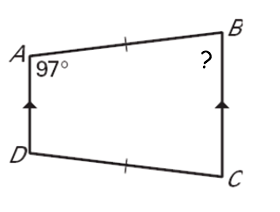Section 4 - Skills Maint 1
Identify *
1 point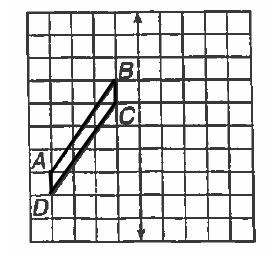Which quad has only ONE set of parallel lines? *
1 point
Trapezoid. Find angle S. *
1 point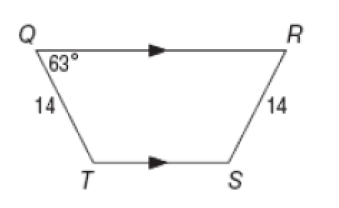solve for x on this trapezoid *
1 point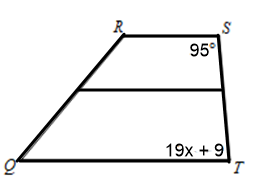Trapezoid. Solve for x. *
1 point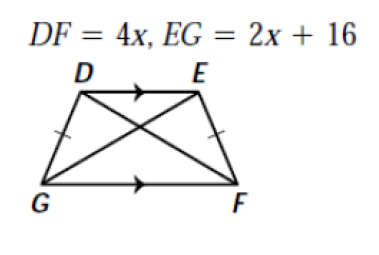MS = B + B/ 2 *
1 point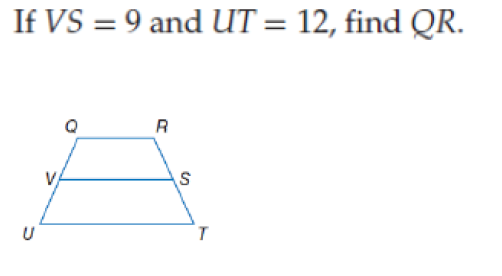Find angle x in this rhombus *
1 point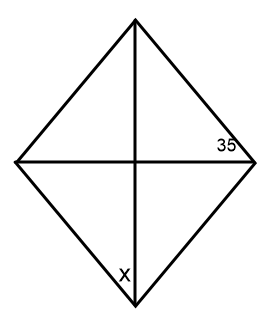Parallelogram *
1 point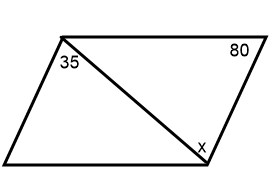Find angle C in this trapezoid *
1 point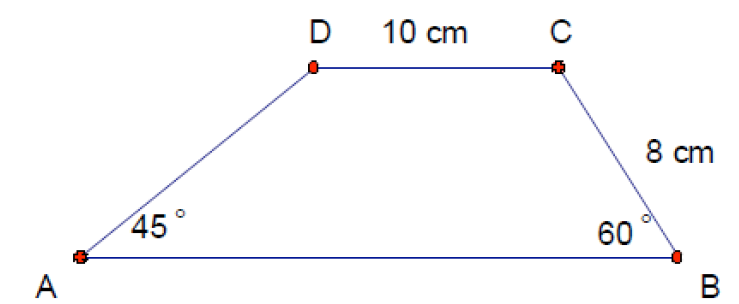Find angle y in this rhombus *
1 point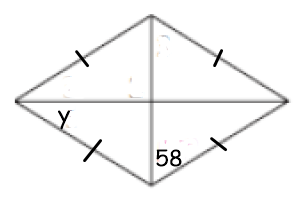Parallelogram. Find x only. *
1 point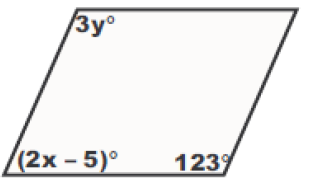Identify *
1 point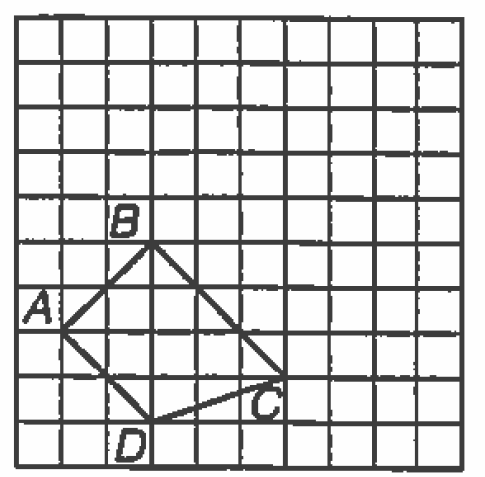A parallelogram has one side of 12 and another of 16. What is the perimeter? *
1 point
Period *
Last Name *
Find x in this parallelogram *
1 point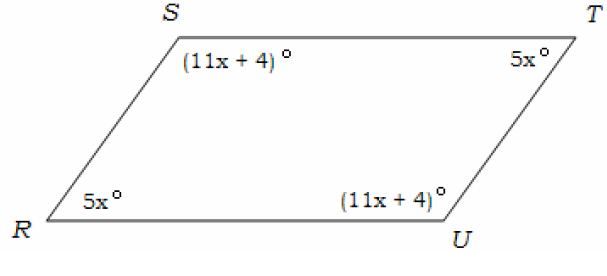Solve for x in this rhombus *
1 point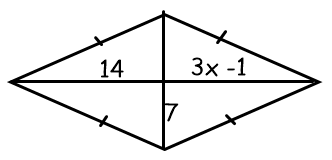Find angle x in this parallelogram *
1 point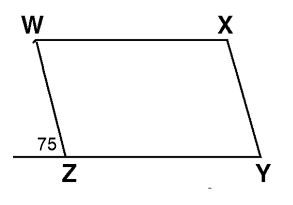Find x on this parallelogram *
1 point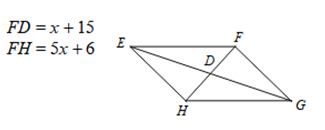Find angle B *
1 point